Noetherian ring

View a complete list of semi-basic definitions on this wiki
This article defines a property of commutative unital rings; a property that can be evaluated for a commutative unital ring
View all properties of commutative unital rings
VIEW RELATED: Commutative unital ring property implications | Commutative unital ring property non-implications |Commutative unital ring metaproperty satisfactions | Commutative unital ring metaproperty dissatisfactions | Commutative unital ring property satisfactions | Commutative unital ring property dissatisfactions

Definition

Below are some equivalent definitions of Noetherian ring:

No. Shorthand A commutative unital ring is termed Noetherian if ... A commutative unital ring$R$ is termed Noetherian if ...
1 ascending chain condition on ideals any ascending chain of ideals stabilizes after a finite length arbitrary version: if$J$ is a well-ordered set and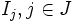$I_j, j \in J$ are ideals such that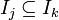$I_j \subseteq I_k$ for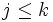$j \le k$, there exists some$j \in J$ such that$I_j = I_k$ for all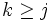$k \ge j$.
countable chain version: If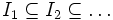$I_1 \subseteq I_2 \subseteq \dots$ is an ascending chain of ideals, then there exists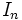$I_n$ such that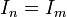$I_n = I_m$ for all$m \ge n$.
2 finite generation of ideals every ideal in the ring is finitely generated. for every ideal$I$ in$R$, there exist elements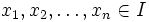$x_1, x_2, \dots, x_n \in I$ such that$I$ is the ideal generated by$x_1, x_2, \dots, x_n$.
3 finite generation of prime ideals every prime ideal in the ring is finitely generated. for every prime ideal$P$ in$R$, there exist elements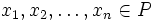$x_1, x_2, \dots, x_n \in P$ such that$P$ equals the ideal generated by$x_1, x_2, \dots, x_n$.

Equivalence of definitions

Further information: equivalence of definitions of Noetherian ring

Relation with other properties

Conjunction with other properties

Noetherian domain integral domain
reduced Noetherian ring reduced ring: it has no nonzero nilpotent elements.
Noetherian normal domain normal domain
Noetherian unique factorization domain unique factorization domain
local Noetherian ring local ring
local Noetherian domain local domain
zero-dimensional Noetherian ring zero-dimensional ring: every prime ideal in it is a maximal ideal
one-dimensional Noetherian domain one-dimensional domain: integral domain in which every nonzero prime ideal is maximal
finite-dimensional Noetherian ring finite-dimensional ring: its Krull dimension is finite.

Stronger properties

Property Meaning Proof of implication Proof of strictness (reverse implication failure) Intermediate notions
Polynomial ring over a field$k[x]$ for a field$k$ click here
Artinian ring descending chain of ideals stabilizes eventually Artinian implies Noetherian Noetherian not implies Artinian click here
Principal ideal ring every ideal is principal principal ideal ring implies Noetherian Noetherian not implies principal ideal ring click here

Weaker properties

Property Meaning Proof of implication Proof of strictness (reverse implication failure) Intermediate notions

Metaproperties

Closure under taking the polynomial ring

This property of commutative unital rings is polynomial-closed: it is closed under the operation of taking the polynomial ring. In other words, if$R$ is a commutative unital ring satisfying the property, so is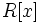$R[x]$

View other polynomial-closed properties of commutative unital rings

The polynomial ring over a Noetherian ring is again Noetherian. This is a general formulation of the Hilbert basis theorem, which asserts in particular that the polynomial ring over a field is Noetherian. Further information: Noetherianness is polynomial-closed

Closure under taking quotient rings

This property of commutative unital rings is quotient-closed: the quotient ring of any ring with this property, by any ideal in it, also has this property

View other quotient-closed properties of commutative unital rings

The quotient ring of a Noetherian ring by an ideal, is also Noetherian. Further information: Noetherianness is quotient-closed

Closure under taking subrings

This property of commutative unital rings is not closed under taking subrings; in other words, a subring of a commutative unital ring with this property need not have this property

A subring of a Noetherian ring is not necessarily Noetherian. For this, consider any non-Noetherian integral domain; this is a subring of a field, which is Noetherian.

Closure under taking localizations

This property of commutative unital rings is closed under taking localizations: the localization at a multiplicatively closed subset of a commutative unital ring with this property, also has this property. In particular, the localization at a prime ideal, and the localization at a maximal ideal, have the property.
View other localization-closed properties of commutative unital rings

A localization of a Noetherian ring is Noetherian. Intuitively, when we take localizations, we land up with fewer ideals, so the ascending chain condition becomes easier to satisfy. Further information: Noetherianness is localization-closed

Direct products

This property of commutative unital rings is finite direct product-closed: a finite direct product of rings with this property, also has this property
View other finite direct product-closed properties of commutative unital rings

A direct product of finitely many Noetherian rings is again Noetherian. This is essentially because ideals in the direct product look like direct products of ideals in the factors. For full proof, refer: Noetherianness is finite direct product-closed

Closure under taking completions

This property of commutative unital rings is completion-closed: the completion of a ring with this property, at any maximal ideal, also has this property
View other completion-closed properties of commutative unital rings

The completion of a Noetherian ring at a maximal ideal is again a Noetherian ring. For full proof, refer: Noetherianness is completion-closed